# The Power of IPython Notebook + Pandas + and Scikit-learn

##### I did a project with some nice tools, and now post this to recommend those tools | June 10, 2016

IPython Notebook, Numpy, Pandas, MongoDB, R — for the better part of a year now, I have been trying out these technologies as part of Udacity’s Data Analyst Nanodegree. My undergrad education barely touched on data visualization or more broadly data science, and so I figured being exposed to the aforementioned technologies would be fun. And fun it has been, with R’s powerful IDE-powered data mundging and visualization techniques having been particularly revelatory. I learned enough of R to create some complex visualizations, and was impressed by how easy is to import data into its Dataframe representations and then transform and visualize that data. I also thought RStudio’s paradigm of continuously intermixed code editing and execution was superior to my habitual workflow of just endlessly cycling between tweaking and executing of Python scripts.

Still, R is a not-quite-general-purpose-language and I hit upon multiple instances in which simple things were hard to do. In such times, I could not help but miss the powers of Python, a language I have tons of experience with and which is about as general purpose as it gets. Luckily, the courses also covered the equivalent of an R implementation for Python: the Python Data Analysis Library, Pandas. This let me use the features of R I now liked — dataframes, powerful plotting methods, elegant methods for transforming data — with Python’s lovely syntax and libraries I already knew and loved. And soon I got to do just that, using both Pandas and the supremely good Machine Learning package Scikit-learn for the final project of Udacity’s Intro to Machine Learning Course. Not only that, but I also used IPython Notebook for RStudio-esque intermixed code editing and execution and nice PDF output.

I had such a nice experience with this combination of tools that I decided to dedicate a post to it, and what follows is mostly a summation of that experience. Reading it should be sufficient to get a general idea for why these tools are useful, whereas a much more detailed introdution and tutorial for Pandas can be found elsewhere (for instance here). Incidentally, this whole post was written in IPython Notebook and the source of that can be found here with the produced HTML here.

## Data Summarization

First, a bit about the project. The task was to first explore and clean a given dataset, and then train classification models using it. The dataset contained dozens of features about roughly 150 important employees from the notoriously corrupt company Enron, witch were classified as either a “Person of Interest” or not based on the outcome of investigations into Enron’s corruption. It’s a tiny dataset and not what I would have chosen, but such were the instructions. The data was provided in a bunch of Python dictionaries, and at first I just used a Python script to change it into a CSV and started exploring it in RStudio. But, it soon dawned on me that I would be much better off just working entirely in Python, and the following code is taken verbatim from my final project submission.

And so, the code. Following some imports and a ‘%matplotlib notebook’ comment to allow plotting within IPython, I loaded the data using pickle and printed out some basic things about it (not yet using Pandas):

``````import matplotlib.pyplot as plt
import matplotlib
import pickle
import pandas as pd
import numpy as np
from IPython.display import display
%matplotlib notebook``````
``````enron_data = pickle.load(open("./ud120-projects/final_project/final_project_dataset.pkl", "rb"))

print("Number of people: %d"%len(enron_data.keys()))
print("Number of features per person: %d"%len(list(enron_data.values())))
print("Number of POI: %d"%sum([1 if x['poi'] else 0 for x in enron_data.values()]))``````
``````Number of people: 146
Number of features per person: 21
Number of POI: 18
``````

But working with this set of dictionaries would not be nearly as fast or easy as a Pandas dataframe, so I soon converted it to that and went ahead and summarized all the features with a single method call:

``````df = pd.DataFrame.from_dict(enron_data)
del df['TOTAL']
df = df.transpose()

numeric_df = df.apply(pd.to_numeric, errors='coerce')

numeric_df.describe()``````
bonus deferral_payments deferred_income director_fees exercised_stock_options expenses from_messages from_poi_to_this_person from_this_person_to_poi loan_advances long_term_incentive other poi restricted_stock restricted_stock_deferred salary shared_receipt_with_poi to_messages total_payments total_stock_value
count 81.000000 38.000000 48.000000 16.000000 101.000000 94.000000 86.000000 86.000000 86.000000 3.000000 65.000000 92.000000 145 109.000000 17.000000 94.000000 86.000000 86.000000 1.240000e+02 125.000000
mean 1201773.074074 841602.526316 -581049.812500 89822.875000 2959559.257426 54192.010638 608.790698 64.895349 41.232558 27975000.000000 746491.200000 465276.663043 0.124138 1147424.091743 621892.823529 284087.542553 1176.465116 2073.860465 2.623421e+06 3352073.024000
std 1441679.438330 1289322.626180 942076.402972 41112.700735 5499449.598994 46108.377454 1841.033949 86.979244 100.073111 46382560.030684 862917.421568 1389719.064851 0.330882 2249770.356903 3845528.349509 177131.115377 1178.317641 2582.700981 9.488106e+06 6532883.097201
min 70000.000000 -102500.000000 -3504386.000000 3285.000000 3285.000000 148.000000 12.000000 0.000000 0.000000 400000.000000 69223.000000 2.000000 False -2604490.000000 -1787380.000000 477.000000 2.000000 57.000000 1.480000e+02 -44093.000000
25% 425000.000000 79644.500000 -611209.250000 83674.500000 506765.000000 22479.000000 22.750000 10.000000 1.000000 1200000.000000 275000.000000 1209.000000 0 252055.000000 -329825.000000 211802.000000 249.750000 541.250000 3.863802e+05 494136.000000
50% 750000.000000 221063.500000 -151927.000000 106164.500000 1297049.000000 46547.500000 41.000000 35.000000 8.000000 2000000.000000 422158.000000 51984.500000 0 441096.000000 -140264.000000 258741.000000 740.500000 1211.000000 1.100246e+06 1095040.000000
75% 1200000.000000 867211.250000 -37926.000000 112815.000000 2542813.000000 78408.500000 145.500000 72.250000 24.750000 41762500.000000 831809.000000 357577.250000 0 985032.000000 -72419.000000 308606.500000 1888.250000 2634.750000 2.084663e+06 2606763.000000
max 8000000.000000 6426990.000000 -833.000000 137864.000000 34348384.000000 228763.000000 14368.000000 528.000000 609.000000 81525000.000000 5145434.000000 10359729.000000 True 14761694.000000 15456290.000000 1111258.000000 5521.000000 15149.000000 1.035598e+08 49110078.000000

This high level summarization of data is one example of what Pandas can do for you. But the main strength is in how easy it is to manipulate the data and derive new things from it. The project instructed me to first summarize some things about the data, and then handle outliers. The summary indicated a large standard deviation for many of the features, and also a lot of missing values in the data for various features. First I dropped features with almost no non-null values, such as loan_advances and restricted_stock_deferred. Then, in order to investigate if any features are particularly bad in terms of outliers, I went ahead computed the standard deviation of each feature for each entry in the data, and easily got summary statistics for this data as well:

``````del numeric_df['loan_advances']
del numeric_df['restricted_stock_deferred']
del numeric_df['director_fees']

std = numeric_df.apply(lambda x: np.abs(x - x.mean()) / x.std())
std = std.fillna(std.mean())
std.describe()``````
bonus deferral_payments deferred_income exercised_stock_options expenses from_messages from_poi_to_this_person from_this_person_to_poi long_term_incentive other poi restricted_stock salary shared_receipt_with_poi to_messages total_payments total_stock_value
count 145.000000 145.000000 145.000000 145.000000 145.000000 145.000000 145.000000 145.000000 145.000000 145.000000 145.000000 145.000000 145.000000 145.000000 145.000000 145.000000 145.000000
mean 0.612134 0.670659 0.690552 0.558364 0.739307 0.487468 0.694769 0.532234 0.670577 0.444004 0.657200 0.525893 0.568830 0.794256 0.648079 0.287221 0.547885
std 0.587181 0.371822 0.409188 0.689763 0.537626 0.669599 0.549542 0.648923 0.491393 0.711333 0.751724 0.735294 0.659254 0.462087 0.582615 0.884946 0.774945
min 0.001230 0.001025 0.002415 0.040311 0.005314 0.028674 0.010294 0.032302 0.027083 0.000058 0.375173 0.044846 0.025148 0.037736 0.041484 0.003077 0.014143
25% 0.380270 0.670659 0.611358 0.346078 0.510059 0.310038 0.481671 0.342075 0.546392 0.297679 0.375173 0.302841 0.250755 0.605495 0.455283 0.130231 0.296228
50% 0.612134 0.670659 0.690552 0.470558 0.739307 0.324161 0.694769 0.412024 0.670577 0.334411 0.375173 0.417338 0.568830 0.794256 0.648079 0.196170 0.423551
75% 0.612134 0.670659 0.690552 0.558364 0.817162 0.487468 0.694769 0.532234 0.670577 0.444004 0.375173 0.525893 0.568830 0.847365 0.648079 0.271301 0.508700
max 4.715491 4.332032 3.103078 5.707630 3.786101 7.473631 5.324312 5.673526 5.097756 7.119750 2.647054 6.051404 4.669820 3.687066 5.062584 10.638201 7.004259

This result suggested that most features have large outliers (larger than 3 standard deviations). In order to be careful not to remove any useful data, I manually inspected all rows with large outliers to see any values that seem appropriate for removal:

``````outliers = std.apply(lambda x: x > 5).any(axis=1)
outlier_df = pd.DataFrame(index=numeric_df[outliers].index)
for col in numeric_df.columns:
outlier_df[str((col,col+'_std'))] = list(zip(numeric_df[outliers][col],std[outliers][col]))
display(outlier_df)
numeric_df.drop('FREVERT MARK A',inplace=True)
df.drop('FREVERT MARK A',inplace=True)``````
('bonus', 'bonus_std') ('deferral_payments', 'deferral_payments_std') ('deferred_income', 'deferred_income_std') ('exercised_stock_options', 'exercised_stock_options_std') ('expenses', 'expenses_std') ('from_messages', 'from_messages_std') ('from_poi_to_this_person', 'from_poi_to_this_person_std') ('from_this_person_to_poi', 'from_this_person_to_poi_std') ('long_term_incentive', 'long_term_incentive_std') ('other', 'other_std') ('poi', 'poi_std') ('restricted_stock', 'restricted_stock_std') ('salary', 'salary_std') ('shared_receipt_with_poi', 'shared_receipt_with_poi_std') ('to_messages', 'to_messages_std') ('total_payments', 'total_payments_std') ('total_stock_value', 'total_stock_value_std')
DELAINEY DAVID W (3000000.0, 1.24731398542) (nan, 0.67065886001) (nan, 0.690552246623) (2291113.0, 0.121547846815) (86174.0, 0.6936264325) (3069.0, 1.3363193564) (66.0, 0.0127001697143) (609.0, 5.67352642171) (1294981.0, 0.635622582522) (1661.0, 0.333603873451) (True, 2.64705431598) (1323148.0, 0.078107486712) (365163.0, 0.457714373186) (2097.0, 0.781228126919) (3093.0, 0.394602217763) (4747979.0, 0.22391802188) (3614261.0, 0.0401335784062)
FREVERT MARK A (2000000.0, 0.553678511813) (6426990.0, 4.33203246439) (-3367011.0, 2.95725609803) (10433518.0, 1.35903759241) (86987.0, 0.711258803121) (21.0, 0.319272057897) (242.0, 2.03617142019) (6.0, 0.352068179278) (1617011.0, 1.00881008801) (7427621.0, 5.00989337561) (False, 0.375173052658) (4188667.0, 1.3518014845) (1060932.0, 4.38570296241) (2979.0, 1.5297529467) (3275.0, 0.465071080146) (17252530.0, 1.54183664695) (14622185.0, 1.72513602468)
HIRKO JOSEPH (nan, 0.612134343218) (10259.0, 0.644790923106) (nan, 0.690552246623) (30766064.0, 5.05623412708) (77978.0, 0.515871316129) (nan, 0.487467982744) (nan, 0.694769235346) (nan, 0.532233915598) (nan, 0.670576589457) (2856.0, 0.332743987428) (True, 2.64705431598) (nan, 0.52589323995) (nan, 0.568830375372) (nan, 0.794256482633) (nan, 0.648079292459) (91093.0, 0.266895026444) (30766064.0, 4.19630821004)
KAMINSKI WINCENTY J (400000.0, 0.556138245963) (nan, 0.67065886001) (nan, 0.690552246623) (850010.0, 0.383592797689) (83585.0, 0.637476115725) (14368.0, 7.47363149225) (41.0, 0.274724723819) (171.0, 1.29672636328) (323466.0, 0.490226746415) (4669.0, 0.331439407211) (False, 0.375173052658) (126027.0, 0.454000599932) (275101.0, 0.0507338450054) (583.0, 0.503654613618) (4607.0, 0.980810226817) (1086821.0, 0.161950156636) (976037.0, 0.363704047455)
LAVORATO JOHN J (8000000.0, 4.71549135347) (nan, 0.67065886001) (nan, 0.690552246623) (4158995.0, 0.21810105193) (49537.0, 0.100958023148) (2585.0, 1.07342360688) (528.0, 5.32431220222) (411.0, 3.69497297064) (2035380.0, 1.4936409531) (1552.0, 0.33368230657) (False, 0.375173052658) (1008149.0, 0.0619063591605) (339288.0, 0.311636142127) (3962.0, 2.3639931937) (7259.0, 2.00764222154) (10425757.0, 0.822328102755) (5167144.0, 0.277836132837)
LAY KENNETH L (7000000.0, 4.02185587986) (202911.0, 0.495369827029) (-300000.0, 0.29833016899) (34348384.0, 5.70763022327) (99832.0, 0.98984158372) (36.0, 0.311124462355) (123.0, 0.668028926971) (16.0, 0.252141237305) (3600000.0, 3.30681561025) (10359729.0, 7.11975001798) (True, 2.64705431598) (14761694.0, 6.05140425399) (1072321.0, 4.44999996622) (2411.0, 1.0477097521) (4273.0, 0.851488248598) (103559793.0, 10.6382007936) (49110078.0, 7.00425896119)
MARTIN AMANDA K (nan, 0.612134343218) (85430.0, 0.586488215565) (nan, 0.690552246623) (2070306.0, 0.16169859209) (8211.0, 0.997237664333) (230.0, 0.205748893335) (8.0, 0.654125583284) (0.0, 0.412024344462) (5145434.0, 5.09775639018) (2818454.0, 1.6932755666) (False, 0.375173052658) (nan, 0.52589323995) (349487.0, 0.36921495869) (477.0, 0.593613378808) (1522.0, 0.21367570973) (8407016.0, 0.609562657351) (2070306.0, 0.196202351233)
SHAPIRO RICHARD S (650000.0, 0.382729377561) (nan, 0.67065886001) (nan, 0.690552246623) (607837.0, 0.427628659031) (137767.0, 1.81257710587) (1215.0, 0.329276547308) (74.0, 0.104676135645) (65.0, 0.237500778364) (nan, 0.670576589457) (705.0, 0.33429178227) (False, 0.375173052658) (379164.0, 0.341483782727) (269076.0, 0.0847481963923) (4527.0, 2.84349038551) (15149.0, 5.06258356331) (1057548.0, 0.165035387918) (987001.0, 0.362025768533)
WHITE JR THOMAS E (450000.0, 0.521456472283) (nan, 0.67065886001) (nan, 0.690552246623) (1297049.0, 0.302304844785) (81353.0, 0.58906842664) (nan, 0.487467982744) (nan, 0.694769235346) (nan, 0.532233915598) (nan, 0.670576589457) (1085463.0, 0.446267416662) (False, 0.375173052658) (13847074.0, 5.64486498335) (317543.0, 0.188873972681) (nan, 0.794256482633) (nan, 0.648079292459) (1934359.0, 0.072623789327) (15144123.0, 1.80502999986)

Looking through these, I found one instance of a valid outlier - Mark A. Frevert (CEO of Enron), and removed him from the dataset.

I should emphasize the benefits of doing all this in IPython Notebook. Being able to tweak parts of the code without reexecuting all of it and reloading all the data made iterating on ideas much faster, and iterating on ideas fast is essential for exploratory data analysis and development of machine learned models. It’s no accident that the Matlab IDE and RStudio, both tools commonly used in the sciences for data processing and analysis, have essentially the same structure. I did not understand the benefits of IPython Notebook when I was first made to use it for class assignments in College, but now it has finally dawned on me that it fills the same role as those IDEs and became popular because it is similaly well suited for working with data.

## Feature Visualization, Engineering and Selection

The project also instructed me to choose a set of features, and to engineer some of my own. In order to get an initial idea of possible promising features and how I could use them to create new features, I computed the correlation of each feature to the Person of Interest classification:

``````corr = numeric_df.corr()
print('\nCorrelations between features to POI:\n ' +str(corr['poi']))``````
``````Correlations between features to POI:
bonus                      0.306907
deferral_payments         -0.075632
deferred_income           -0.334810
exercised_stock_options    0.513724
expenses                   0.064293
from_messages             -0.076108
from_poi_to_this_person    0.183128
from_this_person_to_poi    0.111313
long_term_incentive        0.264894
other                      0.174291
poi                        1.000000
restricted_stock           0.232410
salary                     0.323374
shared_receipt_with_poi    0.239932
to_messages                0.061531
total_payments             0.238375
total_stock_value          0.377033
Name: poi, dtype: float64
``````

The results indicated that ‘exercised_stock_options’, ‘total_stock_value’, and ‘bonus’ are the most promising features. Just for fun, I went ahead and plotted these features to see if I could visually verify their significance:

``````numeric_df.hist(column='exercised_stock_options',by='poi',bins=25,sharex=True,sharey=True)
plt.suptitle("exercised_stock_options by POI")``````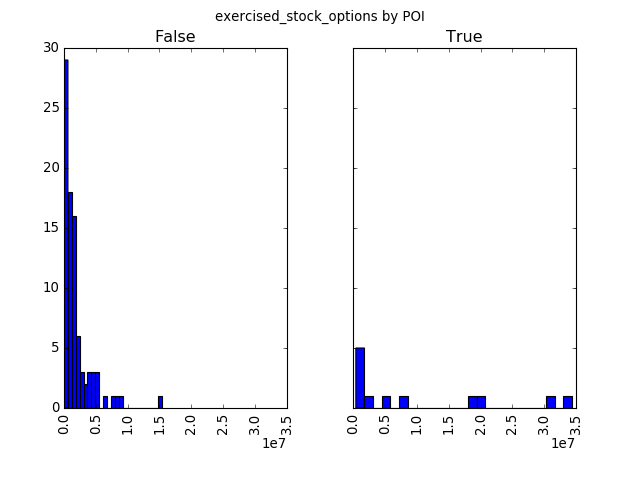``````numeric_df.hist(column='total_stock_value',by='poi',bins=25,sharex=True,sharey=True)
plt.suptitle("total_stock_value by POI")``````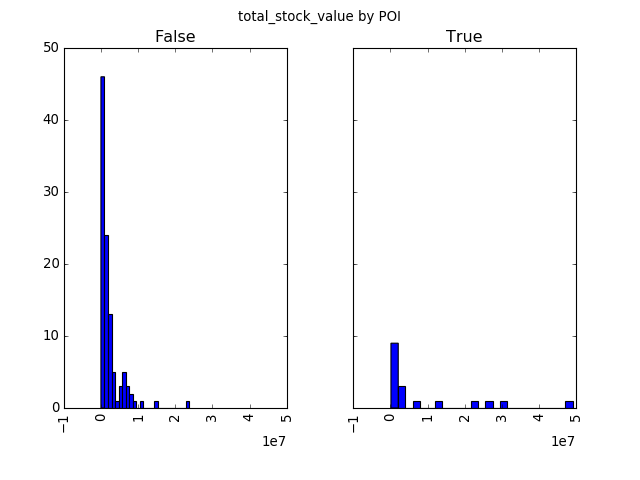``````numeric_df.hist(column='bonus',by='poi',bins=25,sharex=True,sharey=True)
plt.suptitle("bonus by POI")``````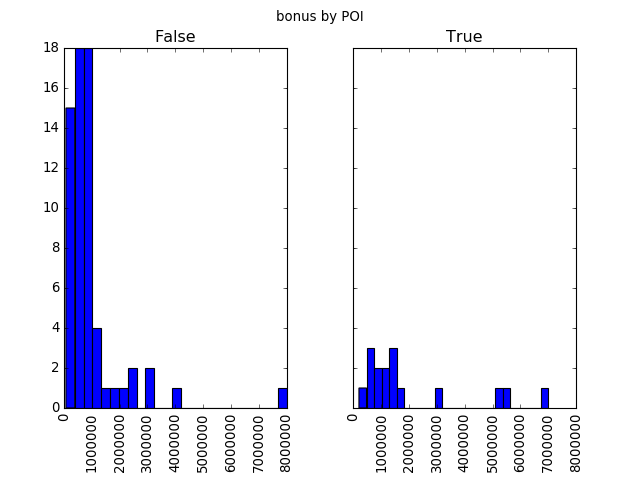As well as one that is not strongly correlated:

``````numeric_df.hist(column='to_messages',by='poi',bins=25,sharex=True,sharey=True)
plt.suptitle("to_messages by POI")``````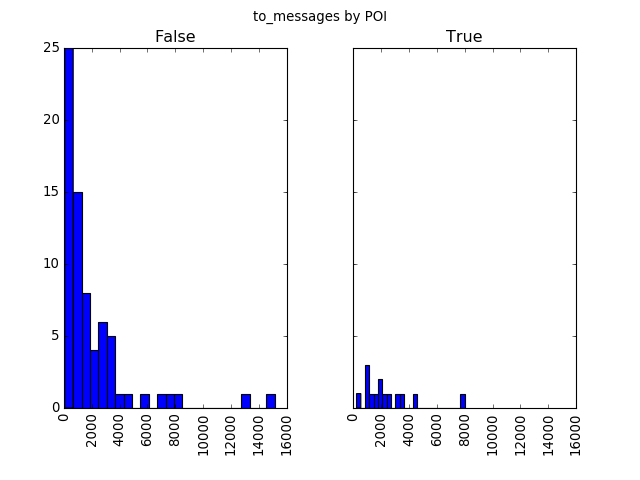The data and plots above indicated that the exercised_stock_options, total_stock_value, and restricted_stock, and to a lesser extent to payment related information (total_payments, salary, bonus, and expenses), are all correlated to Persons of Interest. Therefore, I created new features as sums and ratios of these ones. Working with Pandas made this incredibely easy due to vectorized operations, and though Numpy could similarly make this easy I think Pandas’ Dataframe construct makes it especially easy.

It was also easy to fix any problems with the data before starting to train machine learning models. In order to use the data for evaluation and training, I replaced null values with the mean of each feature so as to be able to use the dataset with Scikit-learn. I also scaled all features to a range of 1-0, to better work with Support Vector Machines:

``````#Get rid of label
del numeric_df['poi']
poi = df['poi']

#Create new features
numeric_df['stock_sum'] = numeric_df['exercised_stock_options'] +\
numeric_df['total_stock_value'] +\
numeric_df['restricted_stock']
numeric_df['stock_ratio'] = numeric_df['exercised_stock_options']/numeric_df['total_stock_value']
numeric_df['money_total'] = numeric_df['salary'] +\
numeric_df['bonus'] -\
numeric_df['expenses']
numeric_df['money_ratio'] = numeric_df['bonus']/numeric_df['salary']
numeric_df['email_ratio'] = numeric_df['from_messages']/(numeric_df['to_messages']+numeric_df['from_messages'])
numeric_df['poi_email_ratio_from'] = numeric_df['from_poi_to_this_person']/numeric_df['to_messages']
numeric_df['poi_email_ratio_to'] = numeric_df['from_this_person_to_poi']/numeric_df['from_messages']

#Feel in NA values with 'marker' value outside range of real values
numeric_df = numeric_df.fillna(numeric_df.mean())

#Scale to 1-0
numeric_df = (numeric_df-numeric_df.min())/(numeric_df.max()-numeric_df.min())``````

Then, I scored features using Scikit-learn’s SelectKBest to get an ordering of them to test with multiple algorithms afterward. Pandas Dataframes can be used directly with Scikit-learn, which is another great benefit of it:

``````from sklearn.feature_selection import SelectKBest
selector = SelectKBest()
selector.fit(numeric_df,poi.tolist())
scores = {numeric_df.columns[i]:selector.scores_[i] for i in range(len(numeric_df.columns))}
sorted_features = sorted(scores,key=scores.get, reverse=True)
for feature in sorted_features:
print('Feature %s has value %f'%(feature,scores[feature]))``````
``````Feature exercised_stock_options has value 30.528310
Feature total_stock_value has value 22.901164
Feature stock_sum has value 16.090150
Feature salary has value 14.428640
Feature poi_email_ratio_to has value 13.619580
Feature bonus has value 11.771121
Feature money_total has value 11.005135
Feature deferred_income has value 9.058555
Feature total_payments has value 8.334006
Feature restricted_stock has value 7.335986
Feature long_term_incentive has value 6.448285
Feature shared_receipt_with_poi has value 6.340473
Feature other has value 4.067974
Feature money_ratio has value 3.781568
Feature from_poi_to_this_person has value 3.626045
Feature email_ratio has value 2.176411
Feature from_this_person_to_poi has value 1.318493
Feature poi_email_ratio_from has value 1.279491
Feature from_messages has value 0.613342
Feature expenses has value 0.543049
Feature to_messages has value 0.400295
Feature deferral_payments has value 0.223368
Feature stock_ratio has value 0.013109
``````

It appeared that several of my features are among the most useful, as ‘poi_email_ratio_to’, ‘stock_sum’, and ‘money_total’ are all ranked highly. But, since the data is so small I had no need to get rid of any of the features and went ahead with testing several classifiers with several sets of features.

# Training and Evaluating Models

Proceding with the project, I selected three algorithms to test and compare: Naive Bayes, Decision Trees, and Support Vector Machines. Naive Bayes is a good baseline for any ML task, and the other two fit well into the task of binary classification with many features and can both be automatically tuned using sklearn classes. A word on SkLearn: it is simply a very well designed Machine Learning toolkit, with great compatibility with Numpy (and therefore also Pandas) and an elegant and smart API structure that makes trying out different models and evaluating features and just about anything one might want short of Deep Learning easy.

I think the code that follows will attest to that. I tested those three algorithms with a variable number of features, from one to all of them ordered by the SelectKBest scoring. Because the data is so small, I could afford an extensive validation scheme and did multiple random splits of the data into training and testing to get an average that best indicated the strength of each algorithm. I also went ahead and evaluated precision and recall besides accuracy, since those were to be the metric of performance. And all it took to do all that is maybe 50 lines of code:

``````from sklearn.naive_bayes import GaussianNB
from sklearn.svm import SVC
from sklearn.grid_search import RandomizedSearchCV, GridSearchCV
from sklearn.tree import DecisionTreeClassifier
from sklearn.metrics import precision_score, recall_score, accuracy_score
from sklearn.cross_validation import StratifiedShuffleSplit
import scipy
import warnings
warnings.filterwarnings('ignore')

gnb_clf = GridSearchCV(GaussianNB(),{})
#No params to tune for for linear bayes, use for convenience

svc_clf = SVC()
svc_search_params = {'C': scipy.stats.expon(scale=1),
'gamma': scipy.stats.expon(scale=.1),
'kernel': ['linear','poly','rbf'],
'class_weight':['balanced',None]}
svc_search = RandomizedSearchCV(svc_clf,
param_distributions=svc_search_params,
n_iter=25)

tree_clf = DecisionTreeClassifier()
tree_search_params = {'criterion':['gini','entropy'],
'max_leaf_nodes':[None,25,50,100,1000],
'min_samples_split':[2,3,4],
'max_features':[0.25,0.5,0.75,1.0]}
tree_search = GridSearchCV(tree_clf,
tree_search_params,
scoring='recall')

search_methods = [gnb_clf,svc_search,tree_search]
average_accuracies = [,,]
average_precision = [,,]
average_recall = [,,]

num_splits = 10
train_split = 0.9
indices = list(StratifiedShuffleSplit(poi.tolist(),
num_splits,
test_size=1-train_split,
random_state=0))

best_features = None
max_score = 0
best_classifier = None
num_features = 0
for num_features in range(1,len(sorted_features)+1):
features = sorted_features[:num_features]
feature_df = numeric_df[features]
for classifier_idx in range(3):
sum_values = [0,0,0]
#Only do parameter search once, too wasteful to do a ton
search_methods[classifier_idx].fit(feature_df.iloc[indices,:],
poi[indices].tolist())
classifier = search_methods[classifier_idx].best_estimator_
for split_idx in range(num_splits):
train_indices, test_indices = indices[split_idx]
train_data = (feature_df.iloc[train_indices,:],poi[train_indices].tolist())
test_data = (feature_df.iloc[test_indices,:],poi[test_indices].tolist())
classifier.fit(train_data,train_data)
predicted = classifier.predict(test_data)
sum_values+=accuracy_score(predicted,test_data)
sum_values+=precision_score(predicted,test_data)
sum_values+=recall_score(predicted,test_data)
avg_acc,avg_prs,avg_recall = [val/num_splits for val in sum_values]
average_accuracies[classifier_idx].append(avg_acc)
average_precision[classifier_idx].append(avg_prs)
average_recall[classifier_idx].append(avg_recall)

score = (avg_prs+avg_recall)/2
if score>max_score and avg_prs>0.3 and avg_recall>0.3:
max_score = score
best_features = features
best_classifier = search_methods[classifier_idx].best_estimator_
print('Best classifier found is %s \n\
with score (recall+precision)/2 of %f\n\
and feature set %s'%(str(best_classifier),max_score,best_features))``````
``````Best classifier found is DecisionTreeClassifier(class_weight=None, criterion='gini', max_depth=None,
max_features=0.25, max_leaf_nodes=25, min_samples_leaf=1,
min_samples_split=2, min_weight_fraction_leaf=0.0,
presort=False, random_state=None, splitter='best')
with score (recall+precision)/2 of 0.370000
and feature set ['exercised_stock_options', 'total_stock_value', 'stock_sum', 'salary', 'poi_email_ratio_to', 'bonus']
``````

Then, I could go right back to Pandas to plot the results. Sure, I could do this with matplotlib just as well, but the flexibility and simplicity of the ‘plot’ function call on a DataFrame is more elegant in my opinion.

``````results = pd.DataFrame.from_dict({'Naive Bayes': average_accuracies,
'SVC':average_accuracies,
'Decision Tree':average_accuracies})
results.plot(xlim=(1,len(sorted_features)-1),ylim=(0,1))
plt.suptitle("Classifier accuracy by # of features")``````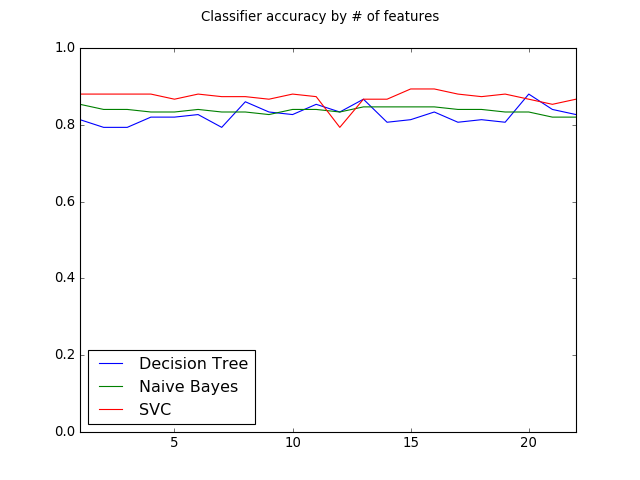``````results = pd.DataFrame.from_dict({'Naive Bayes': average_precision,
'SVC':average_precision,
'Decision Tree':average_precision})
results.plot(xlim=(1,len(sorted_features)-1),ylim=(0,1))
plt.suptitle("Classifier precision by # of features")``````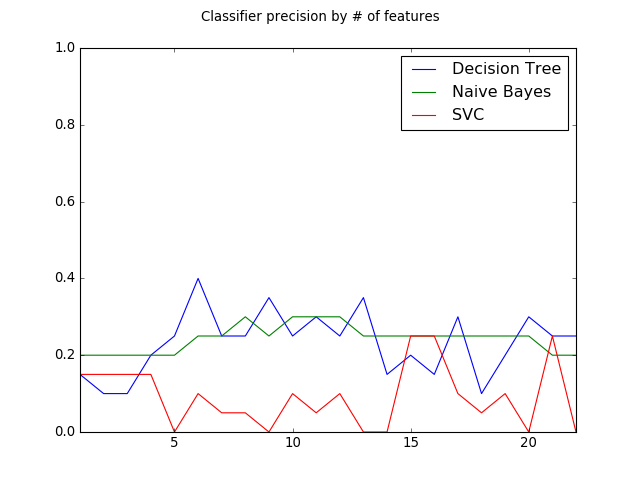``````results = pd.DataFrame.from_dict({'Naive Bayes': average_recall,
'SVC':average_recall,
'Decision Tree':average_recall})
results.plot(xlim=(1,len(sorted_features)-1),ylim=(0,1))
plt.suptitle("Classifier recall by # of features")``````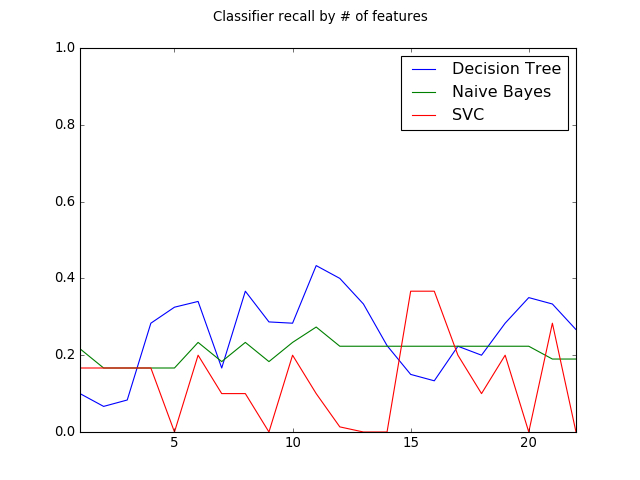As output by my code, the best algorithm was consistently found to be Decision Trees and so I could finally finish up the project by submitting that as my model.

## Conclusion

I did not much care for the project’s dataset and overall structure, but I still greatly enjoyed completing it because of how fun it was to combine Pandas data processing with Scikit-learn model training in the process, with IPython Notebook making that process even more fluid. While not at all a well written introduction or tutorial for these packages, I do hope that this write up about a single project I finished using them might inspire some readers to try out doing that as well.